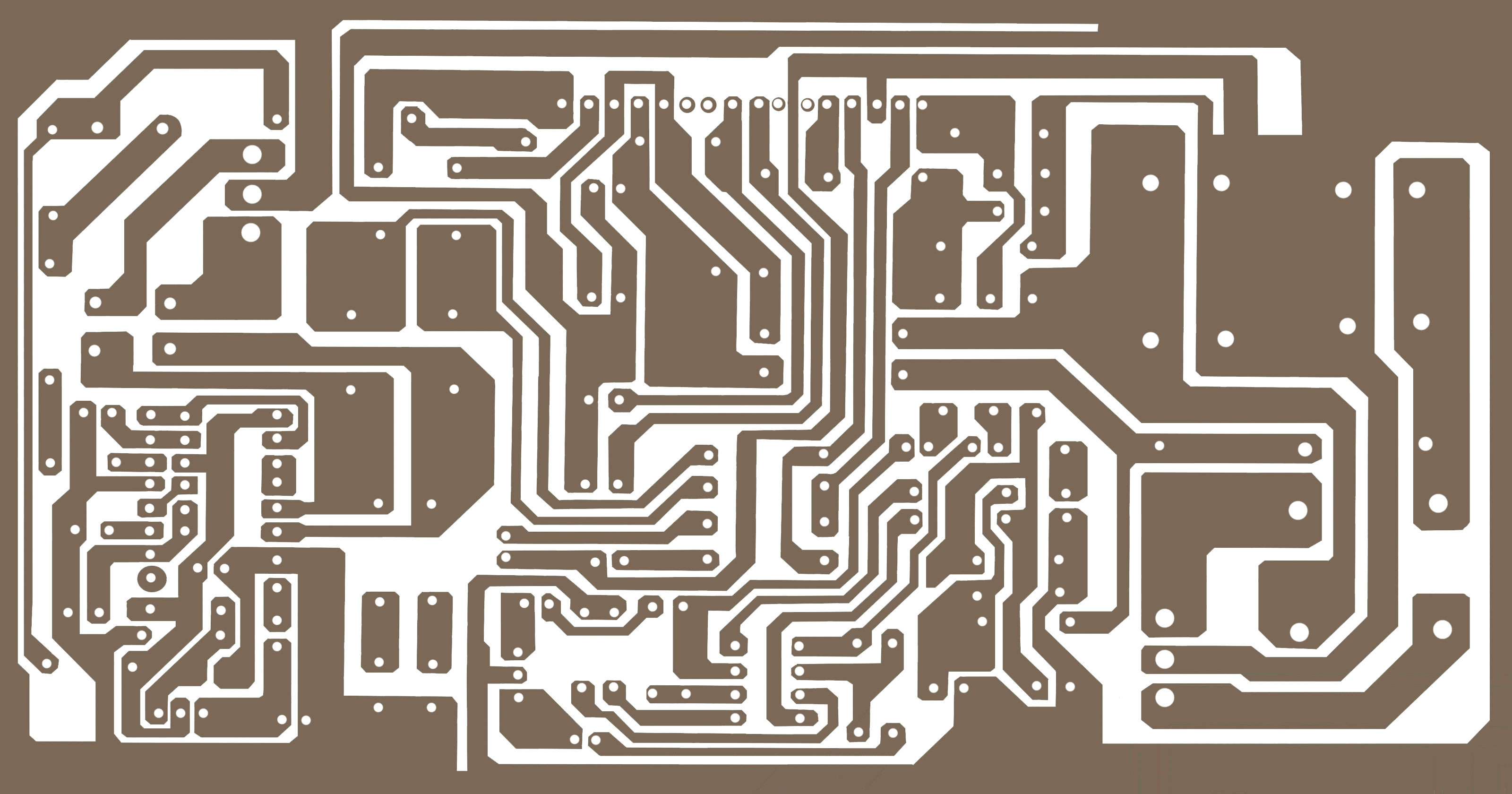Breaking News

# stk amplifier circuit diagram stk401-110

## stk amplifier circuit diagram stk401-110:

This is stk 4191 amplifier circuit diagram stk401-110. here used stk 401-110 ic. this is 70+70 watts amplifier.So 70+70=140 Watts amplifier. for getting 140 watts we have to use 150 watts transformer. If we use 25-0-25 volt so 25+25=50 volt. we know voltage X ampere= Watts. So, 50X3=150 Watts. for 50 volts we have to use 3 amperes. or if we use 30-0-30 volt. then 30+30=60 voltage. So 60X2.5= 150 Watts.

Diagram for this amplifier.Thanks a lot to be with us. If you want another post then please visit our website.

We have another post for you. like as repairing amplifier,